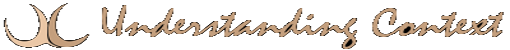# 05 May Learning from Errors

###If at first you don’t succeed, try – try again. Humans are pretty good at learning from our mistakes. In fact, some suggest that whatever doesn’t kill you makes you stronger. Today I’d like to riff on that theme a bit, and talk about ways in which machines can implement learning from errors.

### Error Minimization

One of the amazing advances of neural networks is their ability to learn through repetition. You tell them what the pattern means by explaining the correct output for a given input, and the neural network will adjust its weights in the arcs between nodes until it can consistently deliver the correct output. The formula for learning in a connectionist network was suggested earlier in the reference to Duda and Hart’s work in pattern classification. Learning can be implemented as a process of error minimization. The appeal of this approach in a distributed environment is that local error minimization on the individual neurodes and weights can have a global effect. In fact, this property is probably the major advantage of ANS for perception-related processing. A stimulus is received (a vector is input), and the network feeds it through its layers over weighted arcs. The threshold logic determines the output. Prior to learning, the output will be in error most of the time, but because the actual output can be compared with the desired output, the error e can be calculated.

Understanding Context Cross-Reference
Click on these Links to other posts and glossary/bibliography references
 Prior Post Next Post Multi-Layered Perceptron Impulse Waves in Layers Definitions References Duda 1973  SRI Algorithm SRI Work Anderson 1988 Rosenblatt 1958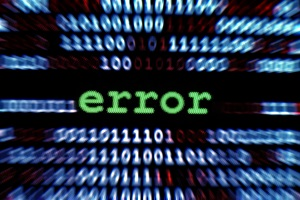Adult learning can often be done through internal or self-correction. We observe that our actions or words do not achieve the desired outcome, so we try something else. For children who have not yet developed the advanced powers of observation and correlation, supervised learning is needed. Someone who knows corrects them. Computers are like little kids – needing constant reinforcement in learning.

Calculated error can be used as the basis for adjusting weights locally to produce the desired output. Because the system is intended to deal with many patterns, rather than forcing the system to the correct output for a single input immediately, weights are adjusted slowly to converge on the correct output. This means that learning requires many cyclic iterations of each input to converge on recognition, but it does enable the learning of many patterns.

### Generalized Delta Rule

A widely used adaptation formula for adjusting weights to converge is called the generalized delta rule, which is shown with the error calculation formula below. The formulae are straightforward. The delta rule is particularly eloquent in its ability, when applied to a distributed network of neurodes with weighted links, to simulate learning and yield a robust, fault-tolerant computing mechanism. Fault-tolerance, in this context, is a function of two things:

1. the network’s ability to recognize patterns despite the presence of noise; and
2. the ability of the network to continue to function despite the failure of some of the neurodes or links in the physical structure.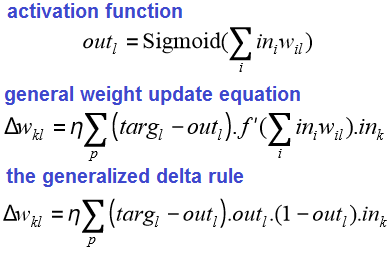On initial input, the output weights will be incorrect. Where “targ” is the target value of output l given pattern k, the following formula calculates the error:

Propagating change formulae back through the network can be a basis for changing weights and permitting the network to learn.

The formula below is the generalized delta rule in which the weights W from neurodes n can be calculated.

An algorithm that uses errors in the output (discreet differences between the correct output and the actual output) to train an artificial neural system to derive a correct output automatically.

Here’s an excerpt from Stanford Research Institute International site explaining a common algorithm for training neural networks:

## A Backpropagation Algorithm

1.
Propagates inputsforward in the usual way,i.e.

• All outputs are computed using sigmoid thresholding of the inner product of the corresponding weight and input vectors.
• All outputs at stage n are connected to all the inputs at stage n+1
2.
Propagates the errors backwards by apportioning them to each unit according to the amount of this error the unit is responsible for.

We now derive the stochastic Backpropagation algorithm for the general case. The derivation is simple, but unfortunately the book-keeping is a little messy.

•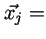input vector for unit j (xji = ith input to the jth unit)
•weight vector for unit j (wji = weight on xji)
•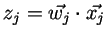, the weighted sum of inputs for unit j
• oj = output of unit j ()
• tj = target for unit j
• Downstream (j) = set of units whose immediate inputs include the output of j
• Outputs = set of output units in the final layer

Since we update after each training example, we can simplify the notation somewhat by imagining that the training set consists of exactly one example and so the error can simply be denoted by E.

We want to calculatefor each input weight wji for each output unit j. Note first that since zj is a function of wji regardless of where in the network unit j is located,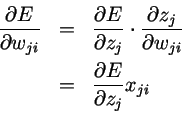Furthermore,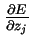is the same regardless of which input weight of unit j we are trying to update. So we denote this quantity by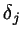.

Consider the case when. We knowSince the outputs of all units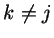are independent of wji, we can drop the summation and consider just the contribution to E by j.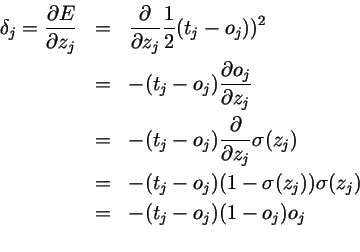ThusNow consider the case when j is a hidden unit. Like before, we make the following two important observations.

1.
For each unit k downstream from jzk is a function of zj
2.
The contribution to error by all units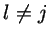in the same layer as j is independent of wji

We want to calculatefor each input weight wji for each hidden unit j. Note that wji influences just zj which influences oj which influenceseach of which influence E. So we can write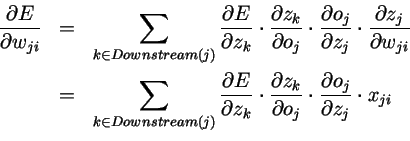Again note that all the terms except xji in the above product are the same regardless of which input weight of unit j we are trying to update. Like before, we denote this common quantity by. Also note that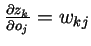and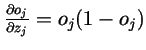. Substituting,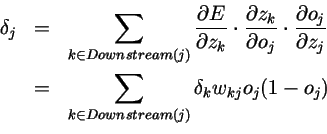Thus,We are now in a position to state the Backpropagation algorithm formally.

Formal statement of the algorithm:

Stochastic Backpropagation (training examples,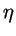ninhno)

Each training example is of the formwhereis the input vector andis the target vector.is the learning rate (e.g., .05). ninh and no are the number of input, hidden and output nodes respectively. Input from unit i to unit j is denoted xji and its weight is denoted by wji.

1. Input the instanceand compute the output ou of every unit.
2. For each output unit k, calculate3. For each hidden unit h, calculate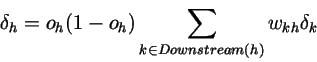4. Update each network weight wji as follows: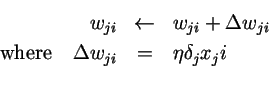For perceptual tasks such as vision (object recognition) and speech recognition, this model works beautifully. It is well suited for many two-dimensional tasks including trend analysis, where the trend can be defined in two-dimensional terms such as trend-line graphs. We will return to this model whenever we encounter tasks of this nature, and effectively use artificial neural networks with learning algorithms such as back propagation.

Click below to look in each Understanding Context section
 Intro Context 1 Brains 2 Neurons 3 Neural Networks 4 Perception and Cognition 5 Fuzzy Logic 6 Language and Dialog 7 Cybernetic Models 8 Apps and Processes 9 The End of Code Glossary Bibliography